Editor Ratings:
User Ratings:
[Total: 0 Average: 0]

Here is a list of 5 free kids calculators. These calculators are specifically meant for kids as they only allow you to perform basic calculations. Unlike other calculators, these calculators only perform basic math operations like multiple, division, addition, subtraction. The main attraction of these calculators for kids are their kid friendly interface. The interface of these calculators has been designed keeping in mind the interest of small kids.

The 5 free kids calculator that I have reviewed in this article are Calculator for kids, Kanakku, Calculator 4 Kids, Children’s Calculator, and Talking Calculator.

## Calculator for Kids: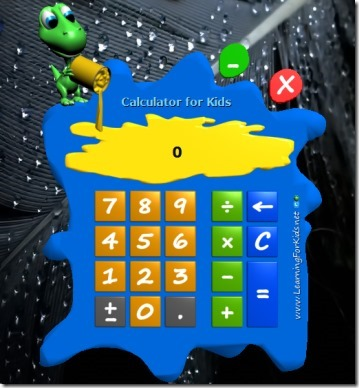Calculator for Kids is the first in the list of free kids calculator. It is a portable calculator that does not require any installation and is also very lightweight ( just 656 KB). The interface of the calculator is very colorful (as you can see in the above screenshot) and suits the style of kids. It helps to generate interest among kids for mathematical operations. The calculator is very simple to use and you can perform calculations like addition, subtraction, multiplication, and division. The most attractive feature of this calculator is its interface. Kids get quickly interested to things they find attractive and that is the USP of this calculator; it can attract kids easily. It looks like colors have been spread all over the place randomly.

## Kanakku: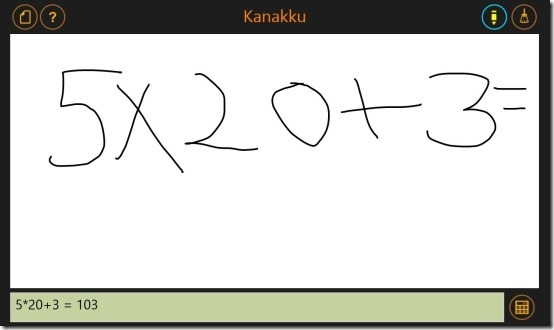Kanakku is the second kids calculator in this list. This kids calculator has been designed for Windows 8 users and is very different from other calculators. There are no keys or numbers on the interface of this calculator. You have to write the numbers and functions that you want to perform, with your own hands. The calculator has a blank interface; you can type your equation in it. The calculator automatically converts your handwritten equation into values and provides its answers. The values and its answer can be seen in the textbox at bottom (shown in the above screenshot). To write a new equation, you can erase the earlier equation with a button given in the top right corner. This calculator allows you to perform only simple calculations like addition, subtraction, division, and multiplication. This is a very good calculator for kids as they can easily write the math problem they are trying to solve.

## Calculator 4 Kids: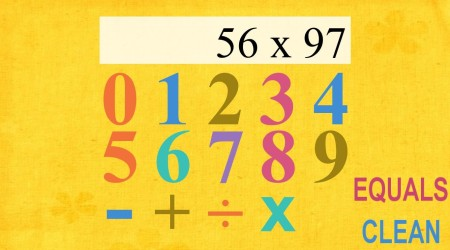Calculating is the third kids calculator in this list. This calculator is, again, made for the users of Windows 8. You can install this lightweight calculator on your system easily, without spending any real time. The interface of this kids’ calculator is so attractive that kids will surely love it. It is a simple calculator with abilities to perform simple mathematical operations like addition, subtraction, multiplication, and division. The interface contains icons for all these operations and you just have to select the one you want to use. Everything has to be done by the click of your mouse and you cannot enter numbers using the keyboard.

## Childrens Calculator: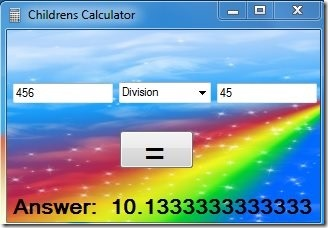Childrens Calculator is the fourth kids calculator in this list. This, again, is a very simple calculator that only performs four basic mathematical operations ( addition, subtraction, multiplication, and division). It is also a very lightweight calculator and will not occupy much space. On the interface of the calculator, there are 3 columns. In the first and third column, you have to type the values that you want. In the middle column, you have to select the operation you want to perform (out of the four operations). After that, you can hit the equal to button and get the answer. The interface of the calculator shows rainbow colors in the background to attract the kids.

## Talking Calculator: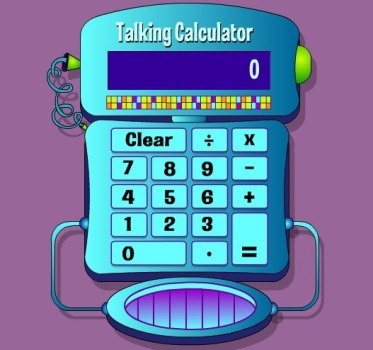The fifth and final kids calculator in this list is Talking Calculator. Basically, it is an online calculator game that comes with a very beautiful interface. The interface has been designed like a telephone and the calculator also talks. As we can judge from the name itself, you can hear all the calculations you do on it. As you press the number keys, the calculator speaks those numbers in real time. This feature is the USP of this calculator and kids are sure to get attracted towards it. The calculator performs basic maths calculations and is specifically meant to be used by kids.

All these five kids calculator allow kids to get more involved in learning simple math calculations. Try them out and let me know which one your kid likes most.

You can also read our earlier article on 5 free scientific calculator software.

 Editor Ratings: User Ratings:[Total: 0 Average: 0] Free/Paid: Free Tags: calculatorkids